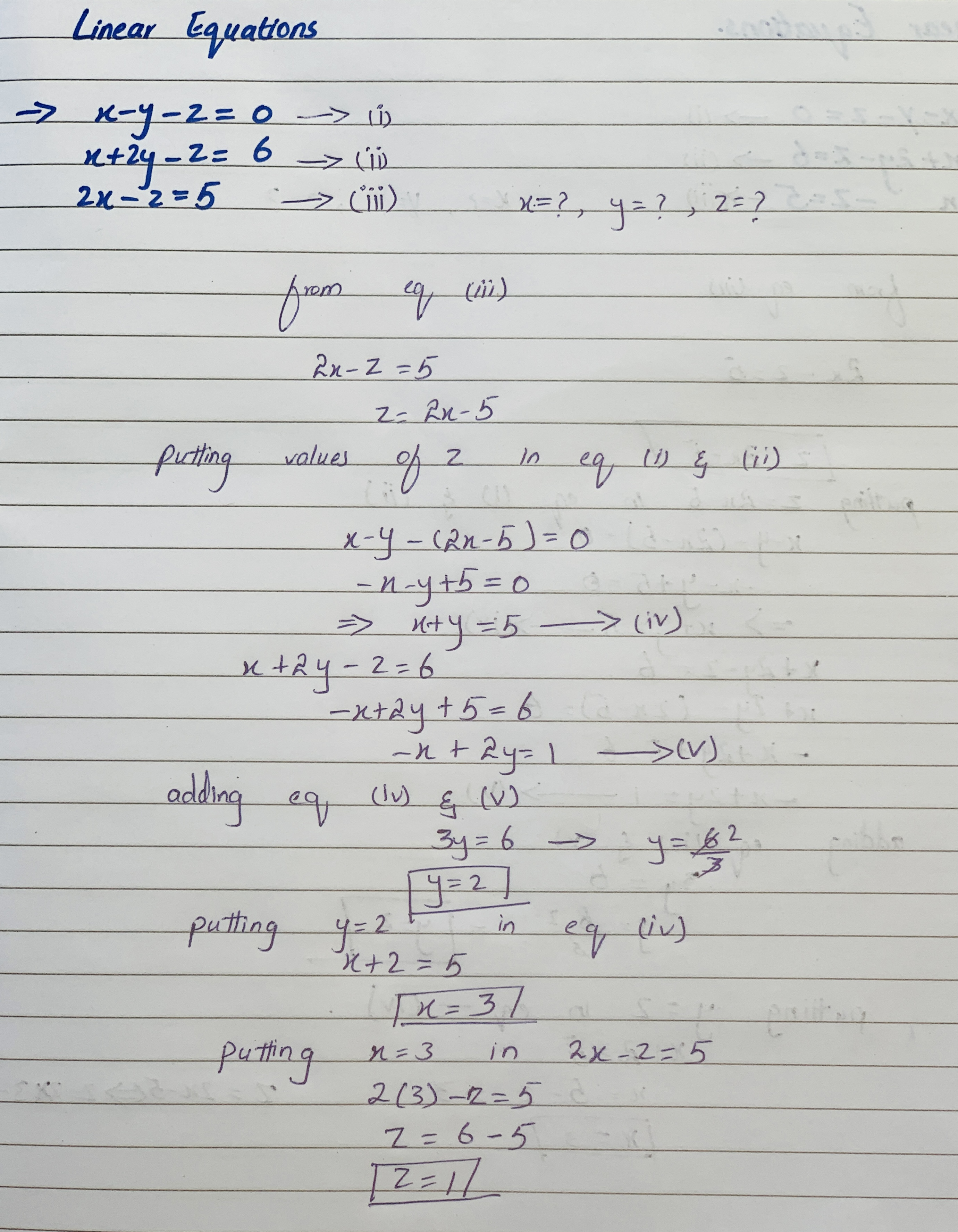Ask question

# Solve linear equation x-y-z=0 x+2y-z=6 2x-z=5# Solve linear equation x-y-z=0 x+2y-z=6 2x-z=5

Question
Forms of linear equationsasked 2021-01-02
Solve linear equation
$$\displaystyle{x}-{y}-{z}={0}$$ PSK $$\displaystyle{x}+{2}{y}-{z}={6}$$
$$\displaystyle{2}{x}-{z}={5}$$

## Answers (1)2021-01-03### Relevant Questionsasked 2021-03-29
Solve the system. If the system does not have one unique solution, also state whether the system is onconsistent or whether the equations are dependent.
2x-y+z=-3
x-3y=2
x+2y+z=-7asked 2020-10-23

Determine if (1,3) is a solution to the given system of linear equations.

$$5x+y=8$$

$$x+2y=5$$asked 2020-10-27

Determine if (1,3)is a solution to given system of linear equations
$$y-2x=3$$
$$3x-2y=5$$asked 2021-03-28
Find all the solutions of the system of equations:
x+2y-z=0, 2x+y+z=0, x-4y+5z=0.asked 2020-11-09
Write the augmented matrix for the system of linear equations
2y-z=7
x+2y+z=17
2x-3y+2z=-1asked 2021-03-15
Use back-substitution to solve the system of linear equations.
$$\displaystyle{b}{e}{g}\in{\left\lbrace{c}{a}{s}{e}{s}\right\rbrace}{x}&-{y}&+{5}{z}&={26}\backslash&\ \ \ {y}&+{2}{z}&={1}\backslash&&\ \ \ \ \ {z}&={6}{e}{n}{d}{\left\lbrace{c}{a}{s}{e}{s}\right\rbrace}$$
(x,y,z)=()asked 2020-11-30
Use Cramer’s Rule to solve (if possible) the system of linear equations.
2x-y=-10
3x+2y=-1asked 2021-03-12

Determine whether the ordered pair is a solution to the given system of linear equations.
(5,3)
$$x-y=2$$
$$x+y=8$$asked 2021-05-09
For the equation, a. Write the value or values of the variable that make a denominator zero. These are the restrictions on the variable. b. Keeping the restrictions in mind, solve the equation.
3/(x + 3) = 5/(2x + 6) + 1/(x - 2)asked 2021-02-19
For the given a system of linear equations
4x+y-5z=8
-2x+3y+z=12
3x-y+4z=5
Use matrix inversion to solve simultaneous equations.
...